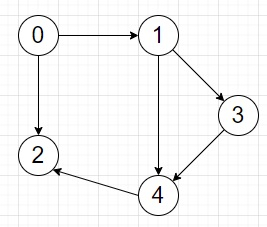# Program to find length of the longest path in a DAG without repeated nodes in Python

PythonServer Side ProgrammingProgramming

Suppose we have one directed acyclic graph represented by the adjacency list. We have to find the longest path in the graph without node repetition.

So, if the input is likethen the output will be 4, as the path is 0 -> 1 -> 3 -> 4 -> 2 with length 4.

To solve this, we will follow these steps −

• ans := 0
• n := node count of graph
• table := a list of size n and fill with -1
• Define a function dfs() . This will take u
• if table[u] is not -1, then
• return table[u]
• p_len := 0
• for each vectex v in graph[u], do
• p_len := maximum of p_len and (1 + dfs(v))
• table[u] := p_len
• return p_len
• From the main method do the following −
• for i in range 0 to n, do
• ans := maximum of ans, dfs(i)
• return ans

## Example (Python)

Let us see the following implementation to get better understanding −

Live Demo

class Solution:
def solve(self, graph):
ans = 0
n = len(graph)
table = [-1] * n
def dfs(u):
if table[u] != -1:
return table[u]
p_len = 0
for v in graph[u]:
p_len = max(p_len, 1 + dfs(v))
table[u] = p_len
return p_len
for i in range(n):
ans = max(ans, dfs(i))
return ans
ob = Solution()
graph = [
[1, 2],
[3, 4],
[],
,
,
]
print(ob.solve(graph))

## Input

graph = [[1, 2],[3, 4],[],,]

## Output

4